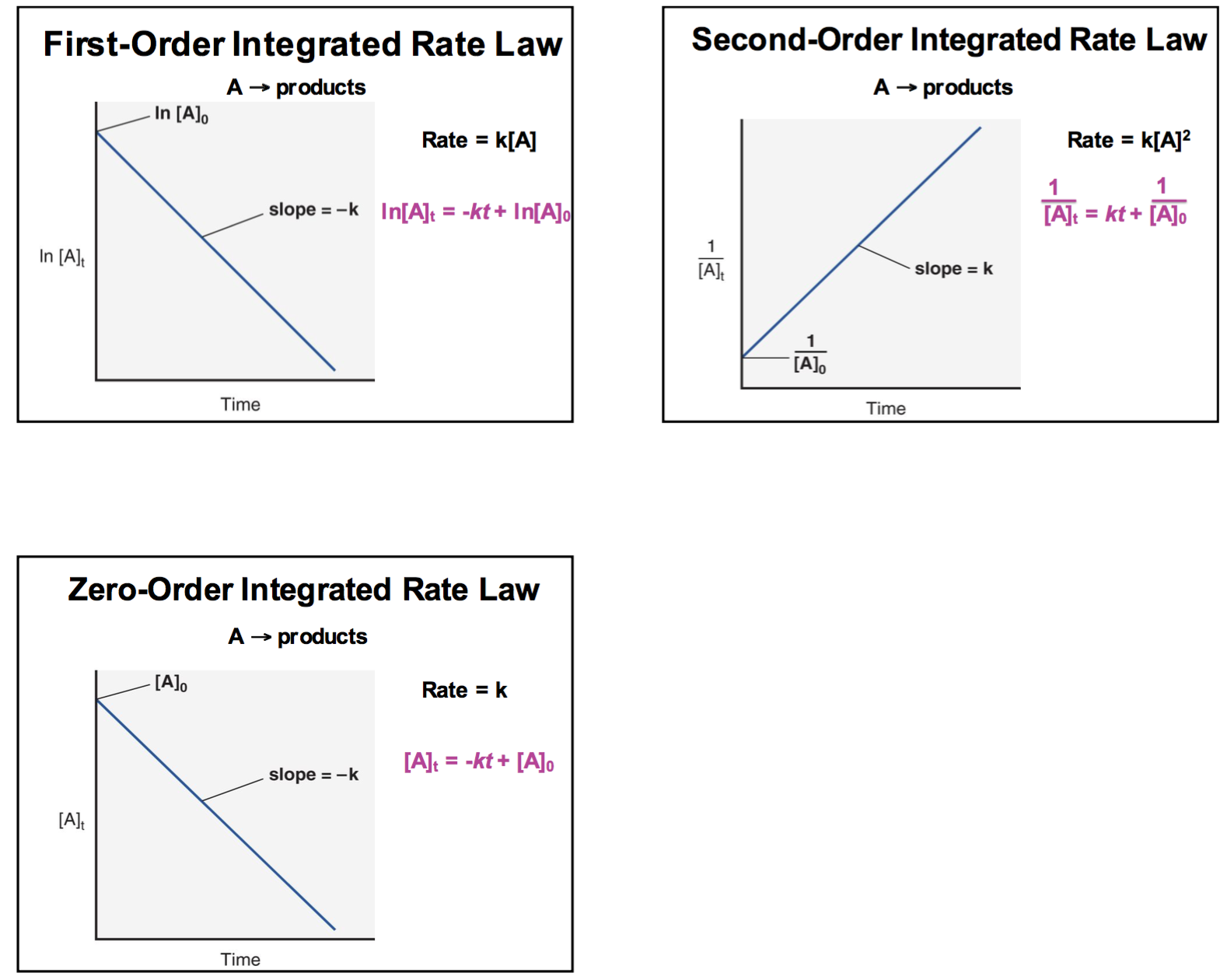Page 1 of 1

### Graphs

Posted: Sun Mar 10, 2019 8:44 pm
Just to clarify, the graph for a first order ln[X] vs time and zero order [X] vs time reaction is a decreasing linear slope. For second order it is an increasing linear slope. Right? So when would they be the increasing and decreasing exponential graphs?

### Re: Graphs

Posted: Sun Mar 10, 2019 9:25 pm
Here is a diagram that you might find helpful:### Re: Graphs

Posted: Sun Mar 10, 2019 10:54 pm
I think that you should always get a linear plot for 0,1,and 2 order reactions!

### Re: Graphs

Posted: Sun Mar 10, 2019 11:21 pm
you should always have a linear graph based on the order of the reaction.

### Re: Graphs

Posted: Sun Mar 10, 2019 11:47 pm
first, second, and zero order reactions have linear plots

### Re: Graphs

Posted: Sun Mar 10, 2019 11:54 pm
All of the graphs for the ordered reactions should have linear plots. It just depends on what is on the axis that determines what is the order of the reaction.

### Re: Graphs

Posted: Mon Mar 11, 2019 2:25 pm
All the graphs will be linear however, the K sign for the slope will be different. Zero and first order graphs will have a negative slope while a second order graph will have a positive K slope.

### Re: Graphs

Posted: Mon Mar 11, 2019 2:33 pm
all graphs are linear but the slopes and axis vary

### Re: Graphs

Posted: Mon Mar 11, 2019 2:39 pm
To my understanding and looking at the textbook first order reactions will have a negative (-) slope, second-order reactions will have a positive (+) slope, and zero order reactions will have a negative (-) slope. This is due to the value of k either being positive or negative which affect the slope of the graph. Hope this helps.